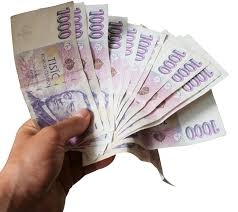# Percent 6567

Jana spent CZK 366 on books, which was 75 percent of her savings. How much CZK did Jana have saved?

x =  488

### Step-by-step explanation:

75/100 x = 366

75/100·x = 366

75x = 36600

x = 36600/75 = 488

x = 488

Our simple equation calculator calculates it.Did you find an error or inaccuracy? Feel free to write us. Thank you!

Tips for related online calculators